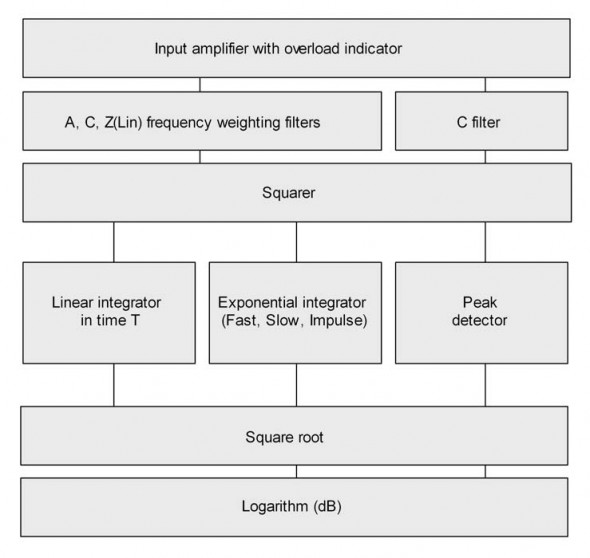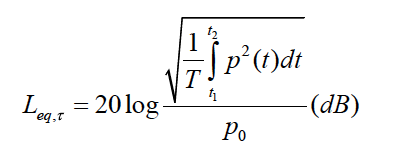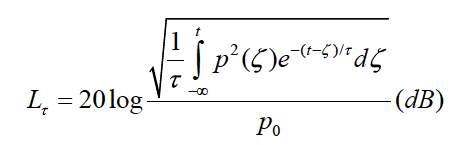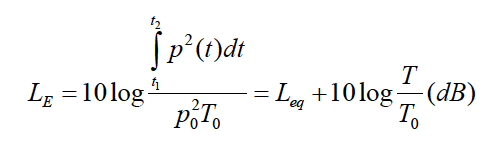# Basic Definitions of Integrating SPL Meters

By Ivo Mateljan

From the ARTA Help files.

## This article will give you some basic definitions of Integrating SPL Meters.

The following functions are available in the ARTA™ virtual SPL meter:

L – Time-weighted Sound Pressure Level (F, S, I)

Lmax – Maximum Time-weighted Sound Pressure Level

Lmin – Minimum Time-weighted Sound Pressure Level

Leq – Time-Average Equivalent Sound Pressure Level

LIeq – Time-Average Impulse-weighted Equivalent Sound Pressure Level

LE – Sound Exposure Level (SEL)

Lpeak – Peak Level

LN – Statistically Calculated Exceedance Level (N = 0.1 99 %)

Implemented frequency weighting filters are: A, C or Z (LIN) weighting.

Implemented time weighting exponential detector are Fast (F), Slow(S) and Impulse (I) type.Figure 9.12 (above) shows block diagram of an integrating SPL meter. The signal from the microphone is going to the input amplifier. An overload indicator shows the state of the input amplifier (or A/D converter in a digital system). The signal from amplifier is going to the frequency weighting filters with three different frequency weighting curves: A, C and Z, as given in Table 9.1 (see Arta Help) and defined in IEC 616721. Letter Z denotes zero-weighting or linear weighting. These weighting curves are used for RMS level measurement. For Peak level measurement C-weighting filter only is used. In the next stage the signal will be squared. The output from a squarer is applied to integrators and peak detector. Finally, after passing square root and logarithm circuits, a sound pressure level in dB will be shown on some display. The following basic values are shown:

Leq – equivalent sound level – is defined as true RMS level obtained by linear integration of squared sound pressure over full measurement time T.where t2-t1 = T is total integration time, p0 is a reference sound pressure 20μPa, p(t) is A, C oz Z frequency weighted sound pressure. Note: If A-weighting filter is applied, then we use label LAeqT or LAT.

In the digital domain this value is obtained by linear averaging samples of squared sound pressure (see. chapter 2.2.1 of Arta Help).

L – time-weighted sound pressure level – is defined for short time intervals with exponential integral:where τ is the exponential function time constant.

Three time constants are denoted with letter F, S, I and are used as:

S – Slow (1000ms)

F – Fast (125ms)

I – Impulse (35 ms, but on falling values a longer time constant of 1500ms is applied)

In the digital domain these values are obtained by applying exponential averaging to every sample of squared time-weighted sound pressure (see. chapter 2.2.1 of Arta Help). For a sine signal between 315Hz and 10kHz all three integrators give the same value as Leq (within 0.1dB), as basically they are all RMS detectors.

The labeling of SPL depends on the applied time and frequency weighting. Generally, we use label:

LXY where X=A, C, Z and Y = F, S, I

for example, LAF is sound pressure level obtained with A – frequency weighting and F – time weighting.

Sometimes SPL-meter is a synonym for a time-weighted sound pressure level meter, as it was applied in the first type of SPL measurement with old analog instrumentation.

LE – sound exposure level (SEL) – is defined as total energy of signal, but expressed as level in one second of time:where T0 = 1s, t2-t1 = T, p0 is a reference pressure 20μPa.

LIeq – impulse-weighted equivalent sound pressure level – is obtained by linear averaging the output of impulse – weighted integrator over measurement time T.

Lpeak – peak level – is obtained every 1s as a peak level on output of C-filter. For sine signals Lpeak is always 3dB larg- er than the output of RMS detectors.

Advanced SPL meters save data of SPL measurements usually every 100ms for Fast time weighting, and every 1 second for other weightings. It enables statistical reports of measured values. A basic report usually gives maximal and minimal value of SPL, maximal peak level and report of time-percentage exceeded levels LN, where N is usually 1%, 5%, 10%, 50%, 90%, 95% and 99%. The meaning of L10 = 87dB is that 10% of time SPL exceeds 87 dB.

Tags: